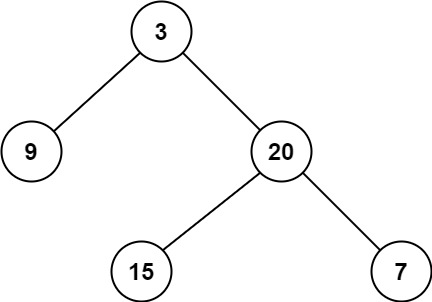# LeetCode: 111. Minimum Depth of Binary Tree

## 题目¶

Given a binary tree, find its minimum depth.

The minimum depth is the number of nodes along the shortest path from the root node down to the nearest leaf node.

Note: A leaf is a node with no children.

Example 1:```Input: root = [3,9,20,null,null,15,7]
Output: 2
```

Example 2:

```Input: root = [2,null,3,null,4,null,5,null,6]
Output: 5
```

Constraints:

• The number of nodes in the tree is in the range [0, 105].
• -1000 <= Node.val <= 1000

## 解法¶

```# Definition for a binary tree node.
# class TreeNode:
#     def __init__(self, val=0, left=None, right=None):
#         self.val = val
#         self.left = left
#         self.right = right
class Solution:
def minDepth(self, root: TreeNode) -> int:
if root is None:
return 0
stack = []
stack.append((root, 1))

while stack:
node, depth = stack.pop(0)
if node.left is None and node.right is None:
return depth

if node.left is not None:
stack.append((node.left, depth + 1))
if node.right is not None:
stack.append((node.right, depth + 1))
```